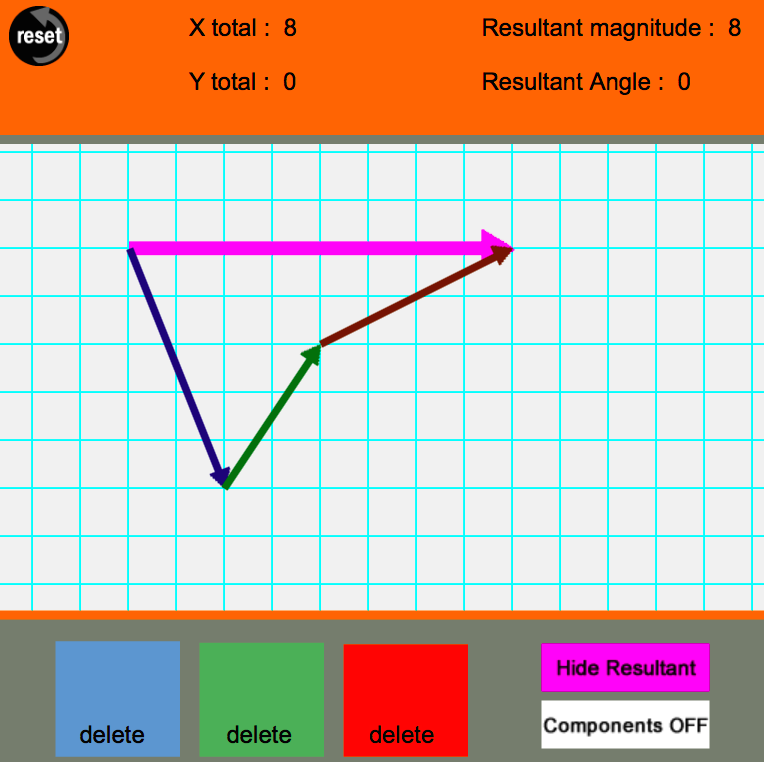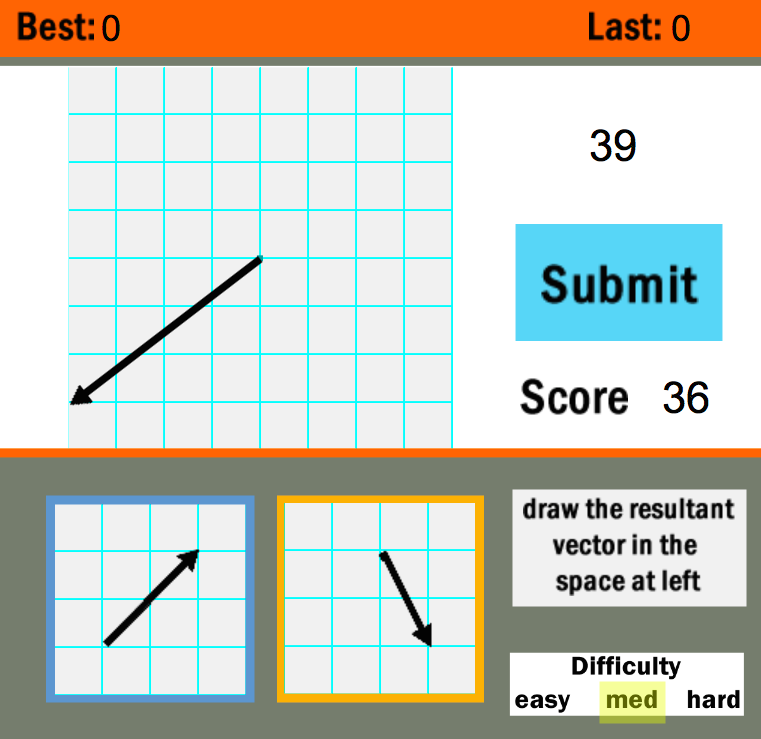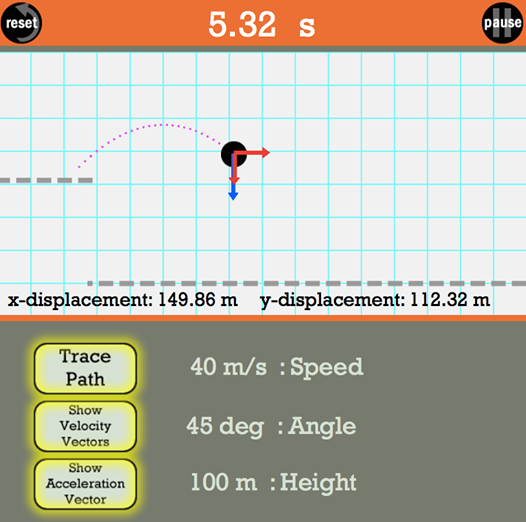Home » Physics » Vectors

# Vectors

### How do we describe this motion?### Look carefully at how the green (horizontal) dots are spaced and how the red (vertical) dots are spaced. What does this spacing tell us?### Allow me to introduce Despicable Me’s Vector### Here’s an object (the black dot) located someplace in space. Note how we can describe it’s position with just three numbers.Image from wikipedia, Coord_planes_color.svg

### For most of this class, just to keep things simple, we’ll consider objects that move in a plane (along a flat surface,) like this:from Wikipedia, Cartesian-coordinate-system.svg

## Apps and interactives

### Newton’s Mountain Canon: Galileo and EinsteinVector Addition

### The Name That Vector Interactive is a skill building tool that presents users with 12 vector addition challenges. Twenty-five vectors are displayed on a grid; each challenge involves adding three of the vectors together to determine the resultant.Vector Guessing Game

### The Vector Addition: Does Order Matter? Investigate with this app

Projectile Simulator## Applications!

### See this lesson from PhysicsClassroom.com Lesson Resolution of Forceshttp://www.physicsclassroom.com/class/vectors/Lesson-3/Resolution-of-Forces

### The Physics of Doing an Ollie on a Skateboard, or, the Science of Why I Can’t SkateImage from real-world-physics-problems .com

### The motion of all particles from a fireworks explosion all trace parabolic paths! Vectors let us analyze this.http://mathforum.org/mathimages/index.php/Envelope

## Related math topics

### How to draw a parabola. For some of the problems that we see here in class you can do the same thing, but have the parabola face in the opposite direction.## Learning Standards

Massachusetts Science Curriculum Framework

1. Motion and Forces: Central Concept: Newton’s laws of motion and gravitation describe and predict the motion of most objects.
1.1 Compare and contrast vector quantities (e.g., displacement, velocity, acceleration force, linear momentum) and scalar quantities (e.g., distance, speed, energy, mass, work).

NGSS

Science and Engineering Practices: Using Mathematics and Computational Thinking

Mathematical and computational thinking in 9–12 builds on K–8 experiences and progresses to using algebraic thinking and analysis, a range of linear and nonlinear functions including trigonometric functions, exponentials and logarithms, and computational tools for statistical analysis to analyze, represent, and model data. Simple computational simulations are created and used based on mathematical models of basic assumptions.

• Apply techniques of algebra and functions to represent and solve scientific and engineering problems.

Although there are differences in how mathematics and computational thinking are applied in science and in engineering, mathematics often brings these two fields together by enabling engineers to apply the mathematical form of scientific theories and by enabling scientists to use powerful information technologies designed by engineers. Both kinds of professionals can thereby accomplish investigations and analyses and build complex models, which might otherwise be out of the question. (NRC Framework, 2012, p. 65)

Students are expected to use mathematics to represent physical variables and their relationships, and to make quantitative predictions. Other applications of mathematics in science and engineering include logic, geometry, and at the highest levels, calculus…. Mathematics is a tool that is key to understanding science. As such, classroom instruction must include critical skills of mathematics. The NGSS displays many of those skills through the performance expectations, but classroom instruction should enhance all of science through the use of quality mathematical and computational thinking.

Common Core Standards for Mathematics (CCSM)

High School: Number and Quantity » Vector & Matrix Quantities. Represent and model with vector quantities.

#### Represent and model with vector quantities.

CCSS.MATH.CONTENT.HSN.VM.A.1
(+) Recognize vector quantities as having both magnitude and direction. Represent vector quantities by directed line segments, and use appropriate symbols for vectors and their magnitudes (e.g., v, |v|, ||v||, v).
CCSS.MATH.CONTENT.HSN.VM.A.2
(+) Find the components of a vector by subtracting the coordinates of an initial point from the coordinates of a terminal point.
CCSS.MATH.CONTENT.HSN.VM.A.3
(+) Solve problems involving velocity and other quantities that can be represented by vectors.

#### Perform operations on vectors.

CCSS.MATH.CONTENT.HSN.VM.B.4
CCSS.MATH.CONTENT.HSN.VM.B.4.A
Add vectors end-to-end, component-wise, and by the parallelogram rule. Understand that the magnitude of a sum of two vectors is typically not the sum of the magnitudes.
CCSS.MATH.CONTENT.HSN.VM.B.4.B
Given two vectors in magnitude and direction form, determine the magnitude and direction of their sum.
CCSS.MATH.CONTENT.HSN.VM.B.4.C
Understand vector subtraction v – w as v + (-w), where –w is the additive inverse of w, with the same magnitude as w and pointing in the opposite direction. Represent vector subtraction graphically by connecting the tips in the appropriate order, and perform vector subtraction component-wise.
CCSS.MATH.CONTENT.HSN.VM.B.5
(+) Multiply a vector by a scalar.
CCSS.MATH.CONTENT.HSN.VM.B.5.A
Represent scalar multiplication graphically by scaling vectors and possibly reversing their direction; perform scalar multiplication component-wise, e.g., as c(vxvy) = (cvxcvy).
CCSS.MATH.CONTENT.HSN.VM.B.5.B
Compute the magnitude of a scalar multiple cv using ||cv|| = |c|v. Compute the direction of cv knowing that when |c|v ≠ 0, the direction of cv is either along v (for c > 0) or against v (for c < 0).
American Association for the Advancement of Science

Operating with Symbols and Equations

• Become fluent in generating equivalent expressions for simple algebraic expressions and in solving linear equations and inequalities.
• Develop fluency operating on polynomials, vectors, and matrices using by-hand operations for the simple cases and using technology for more complex cases.
AAAS Benchmarks (American Association for the Advancement of Science)
9B9-12#2: Symbolic statements can be manipulated by rules of mathematical logic to produce other statements of the same relationship, which may show some interesting aspect more clearly. Symbolic statements can be combined to look for values of variables that will satisfy all of them at the same time.
9B9-12#5: When a relationship is represented in symbols, numbers can be substituted for all but one of the symbols and the possible value of the remaining symbol computed. Sometimes the relationship may be satisfied by one value, sometimes more than one, and sometimes maybe not at all.
12B9-12#2: Find answers to problems by substituting numerical values in simple algebraic formulas and judge whether the answer is reasonable by reviewing the process and checking against typical values.
12B9-12#3: Make up and write out simple algorithms for solving problems that take several steps.
.